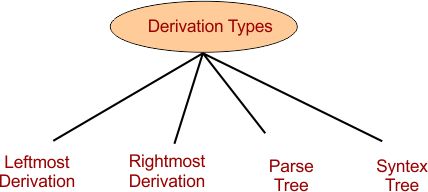Select Page

# Derivation In Automata

The process of deriving a string from grammar is called as derivation in Automata. The
Representation of derivation in the form of tree is called as a  derivation tree.

## Purpose of Derivation

Derivation tree is useful, when a string and grammar (production rules) are given and we have to check whether the string belongs to grammar or not?

## Example of Derivation

As the following production rules are given

S → xB

B → xS| ε

And suppose a string W= xxx.

If the given string is derived from given production rules then derivation and its tree is possible.

## Types of Derivation

There are four basic types derivations in theory of Automata

• Leftmost derivation
• Rightmost derivation
• Parse tree
• Syntax treeBut the first two types of derivations are mostly used.

### Remember

• Leftmost and Rightmost derivation, parse and syntax tree will be similar for unambiguous grammars.
• Leftmost and Rightmost derivation, parse and syntax tree will not be similar For ambiguous grammars,.

Help Other’s By Sharing…